## 2.11Vorticity

Vorticity describes the tendency for a ﬂuid to rotate locally, deﬁned as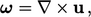(2.37)
where the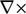operator is the curl derivative. The curl of a vector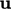is evaluated in Cartesian co-ordinates by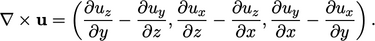(2.38)
The local rotation which vorticity measures is not due to the ﬂow following a curved path. In fact, vorticity is often associated with shear when streamlines may be straight and parallel, i.e. not curved at all.

Vorticity is diﬃcult to picture under shear because the deformation masks the local rotation. By separating the deformation, as in the ﬁgure in Sec. 2.10 , the local rotation is revealed.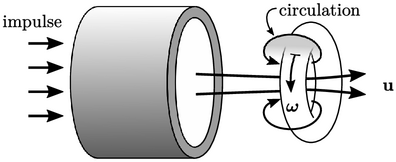Vorticity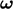is often demonstrated by a vortex ring produced by an air “cannon” with smoke to visualise the ﬂow. It reveals ﬂow circulation around sections of the torus, from front to back. The vorticity vectors are normal to the planes of circulation, along the axis of the torus.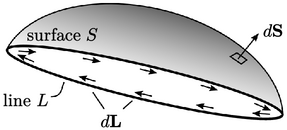Stokes’s theorem8 relates circulation — the integral of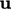around a closed curved line,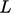— to the integral of vorticity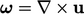over a section of surface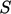bounded by the curve, according to: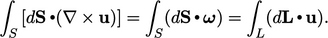(2.39)
In Eq. (2.39 ),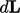is a vector representing a segment of the line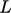. As you stand onlooking in the direction, with your head in the direction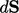,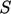is oriented to your left.

Vorticity is related to the spin tensor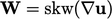in Eq. (2.33 ) by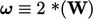, where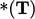is the Hodge dual operator which extracts components of a vector from a tensor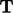as shown below: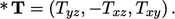(2.40)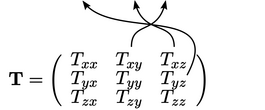The term “spin tensor” emphasises local rotation as opposed to the general path in a similar way that the spin on a ball is clearly distinguished from its trajectory, which curves due to gravity.
8posed by George Stokes as a examination question for the 1854 Smith’s Prize for progress in mathematics and natural philosophy at the University of Cambridge, awarded to James Clerk Maxwell and Edward Routh.

Notes on CFD: General Principles - 2.11 Vorticity